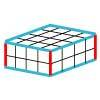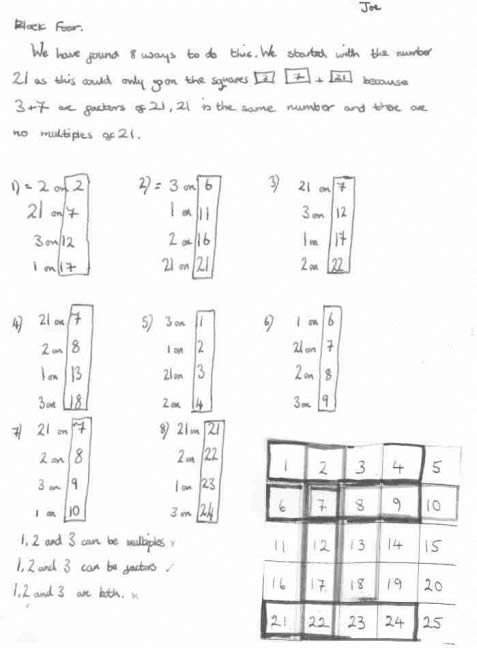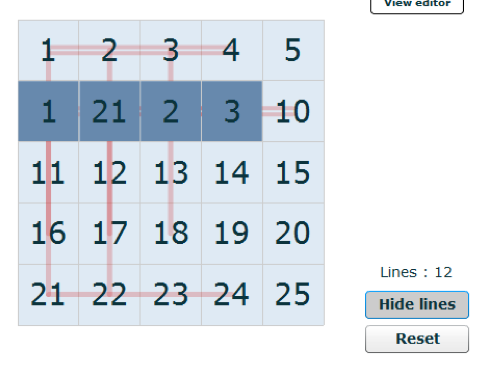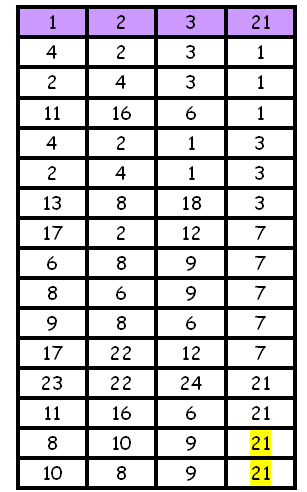#### You may also like### Round and Round the Circle

What happens if you join every second point on this circle? How about every third point? Try with different steps and see if you can predict what will happen.### Making Cuboids

Let's say you can only use two different lengths - 2 units and 4 units. Using just these 2 lengths as the edges how many different cuboids can you make?### Two and Two

How many solutions can you find to this sum? Each of the different letters stands for a different number.

# Factor Lines

##### Age 7 to 14Challenge Level

Joe and Sarah described their solutions very well, although they didn't find all the possible lines of four. Here is Joe's:Hannah wrote to us to tell us about her work - she is still finding possible solutions but says:

I found 12 different solutions. I decided to put the number 21 in place first, then see if we could fit the other numbers on to make the lines. We tried to make diagonal lines, but we couldn't! This was because the number 21 needed to be on 1, 3, 7 or 21 and the other numbers would not create a diagonal line from these.
I like it because it is so much fun try it !
I recorded my lines and the number cards I used on a printed version of the grid. I then decided to change the colour as it was getting confusing to read!

This image shows us Hannah's solutions:Clement Chan from Sha Tin College in Hong Kong has found thirteen ways. He says:

I first tried to find all the possible lines I could make on the grid than looked at each one carefully for example:

I want to make a vertical line from the $1$, I check whether the $21$ can go in anywhere and if it can I check whether the $3$ can go anywhere, and then the $2$ and put the $1$ on to finish the line.

If I want to make a line diagonally from the $5$ then I check it in the same way, and if I want to make a horizontal line from the $15$ I check it the same way etc.

Laquiesha and Chloe from Kingsmoor Primary also thought they had found thirteen ways, but they didn't list them all. They pointed out:

We also found out that you couldn't do diagonals because the no. $21$ had to be placed on the four odd numbers $1$, $3$, $7$, $21$ and you couldn't put the number $2$ in the diagonal because $2$ needs to be on a even number but they were all odd in the diagonal.

(In fact, $2$ could go on the $1$ in the diagonal, couldn't it? But I see what you mean, that you can't then place the other two numbers in the diagonal.) Ben T and Ben H from Brewood Middle School realised that diagonals are impossible too.

Mrs Rankin from Sandown Primary School wrote to say:

A group of children worked together on this investigation today and became very excited when they found fifteen solutions to this problem by using a systematic approach!! (That'??s one more than your solution suggests!)

Well done all of you - you're right. We thought that there were only fourteen solutions. We obviously weren't being as systematic as we thought! Here is a table which shows the group's solutions:I like the way you have organised your recording. I have highlighted the two $21$s in the bottom of your table because I think in fact these should be $7$s. I hope you agree with me.